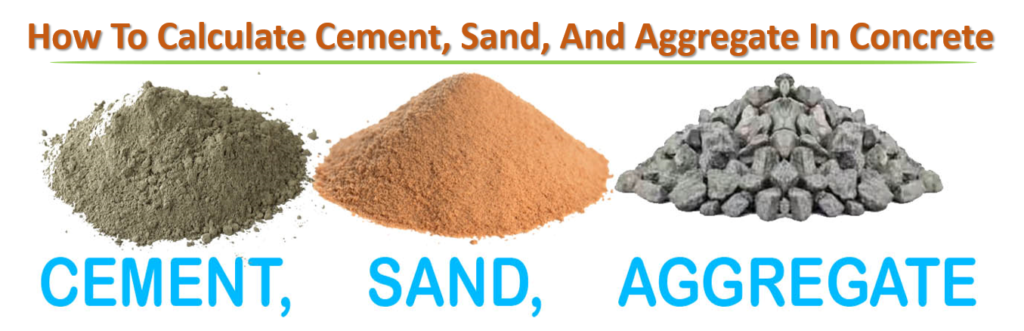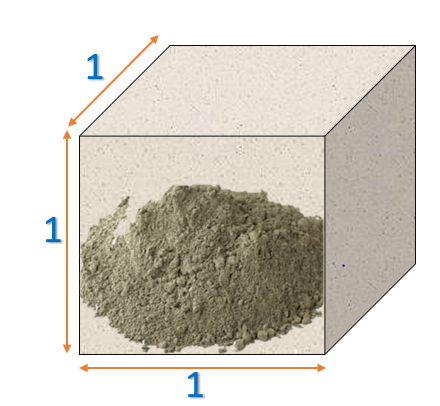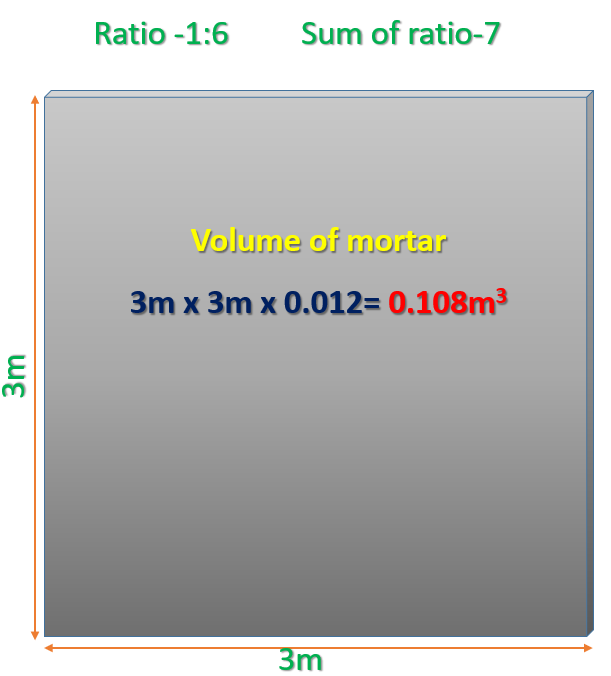# How To Calculate Cement, Sand, & Aggregate Quantity In 1 Cubic metre Concrete## Point Should Be Know Before Estimating

Density of Cement       = 1440 kg/m3
Sand Density                  = 1450-1500 kg/m3
Density of Aggregate   = 1450-1550 kg/m3

How many KG in 1 bag of cement                                 = 50 kg
Cement quantity  in litres in 1 bag of cement          = 34.7 litres
1 Bag of cement in  cubic metres                                  = 0.0347 cubic meter
How many CFT (Cubic Feet)                                            = 1.226 CFT
Numbers of Bags in 1 cubic metre cement               = 28.8 Bags

Specific gravity of cement   = 3.15
Grade of cement                      =  33, 43, 53
Where 33, 43, 53 compressive strength of cement in N/mm2

M-20 = 1 : 1.5 : 3  = 5.5, (Cement : Sand : Aggregate)
Some of Mix is – 5.5

Where, M   = Mix
20  = Characteristic Compressive strength

Consider volume of concrete = 1m3

Dry  Volume of Concrete = 1 x 1.54 = 1.54 m3    (For Dry Volume Multiply By 1.54)

Now we start calculation for find Cement, Sand and Aggregate quality in 1 cubic meter concrete

• #### CALCULATION FOR CEMENT QUANTITYCement=  (1/5.5) x  1.54    = 0.28 m3   1 is a part of cement, 5.5 is sum of ratio
Density of Cement is 1440/m3

= 0.28  x 1440 = 403.2 kg

We know each bag of cement is 50 kg
For Numbers of Bags =   403.2/50     = 8 Bags

We Know in one bag of cement = 1.226 CFT

For Calculate in CFT (Cubic Feet) = 8  x 1.225      = 9.8 Cubic Feet

• #### CALCULATION FOR SAND QUANTITYConsider volume of concrete = 1m3

Dry  Volume of Concrete = 1 x 1.54 = 1.54 m3

Sand=  (1.5/5.5) x 1.54    = 0.42 m3   1.5 is a part of Sand, 5.5 is sum of ratio

Density of Sand is 1450/m3

For KG = 0.42 x 1450 = 609 kg

As we know that 1m3 = 35.31 CFT

For Calculation in Cubic Feet   = 0.42 x 35.31 = 14.83 Cubic Feet

• #### CALCULATION FOR AGGREGATE QUANTITYConsider volume of concrete = 1m3

Dry  Volume of Concrete = 1 x 1.54 = 1.54 m3

Aggregate =   (3/5.5) x 1.54 = 0.84 m3 ∴ 3 is a part of cement, 5.5 is sum of ratio

Density of Aggregate is 1500/m

Calculation for KG = 0.84 x 1500   = 1260 kg

As we know that 1 m3 = 35.31 CFT

Calculation for CFT  = 0.84 x 35.31 = 29.66 Cubic Feet

Also you can Download Excel Sheet for Calculate Concrete Volume (Cement, Sand and Aggregate ) Quantity.Excel Sheel For Concrete Quantity Calculation

### Now we start calculation for find Cement, Sand  quality in mortar  for Plaster

Area of brick wall for plaster = 3m x 3m =9m2Plaster Thickness = 12mm (Outer-20mm, Inner 12mm)

Volume of mortar = 9m2  0.012m            = 0.108m3

Ratio for Plaster Taken is                             = 1 : 6

Sum of ratio is                                                   = 7

Calculation for Cement Volume

Dry Volume of Mortar = 0.108  1.35 = 0.1458 m3

Cement= (1/7) = 0.0208 m3

Density of Cement is 1440/m3

= 0 1440              = 29.99 kg

We know each bag of cement is 50 kg

= (29.99/50)        = 0.599 Bags

Calculation for Sand Volume

Sand = (6/7) x 0.1458      = 0.124m3

Density of Sand is 1450/m3

= 0 1450                = 181.2 kg

Now we find how many CFT (Cubic feet) Required

As we know that 1m3 = 35.31 CFT

= 0.124*35.31

= 4.37 CFT (Cubic Feet)### 29 Responses

1.Mueed Saeed says:

Very helpful in calculations of material. Small contractors can take help for rapid estimation of buildibg material.
Thanks a lot

•Tutorial Tips Civil says:

yes, thank you

2.James says:

Can i get a pdf document with this very helpful information

3.Egide says:

There are very important Knowledge to professional Engineers

4.Maaz Khan says:

Sir you are incredible thanks alotttttttttttttttttttt

5.success says:

Nice write up. Apt and explanatory. thanks so much, keep it up.

•Tutorial Tips Civil says:

Thanks

6.Dilip says:

Very easy calculate and very good thanks …

7.Nebiyu says:

Pleace help me

•Tutorial Tips Civil says:

tell me

8.Nebiyu says:

About cement casting and ration with type of concrit?

9.Ijaz says:

Can we multiply 1.54 cub meter in feet?

10.Farooq Bashir says:

Very useful for estimation and for general calculation.

11.Brentwood Stamped Concrete says:

This is some brilliant information! I’m so impressed that people put such extremely helpful info on the internet. Thank you!

12.Mobile Asphalt Plant says:

This tea can only be plucked for a little more than two months
of throughout March and April. Let the Chinese and
Indians fight it out for dominance (since that’s what both of them want, in any case).

13.bindu says:

brick work me sand cement ki qty excel me kese data sheet banaye?

•Tutorial Tips Civil says:

please go to my brick calculation post there you can get your excel sheet

14.Priyanshu says:

Very informative

15.Matthew A says:

Very helpful and educative. Can have a PDF copy of this very information.

16.MOHAMMED SAKIR says:

Thats good explain i like

17.ephrata tesfaye says:

18.Chamila says:

Very important.. Thanks

19.Abubakar Abdul Afeez says:

Thank you very much for this invaluable breakdown. I have a question, I’m a Nigerian and the mix ratio we use some times get me confused. for example 1:3:6, and 1:2:4 are M15 and M25 respectively, I still see some people saying the latter is M20. Please, I want to really know what is the standard mix ratio. I would also love it if we can get most of your tips in pdf.

Thank you

•Tutorial Tips Civil says:

every country have own IS code: this ration generally follow in India, I don’t know about other country you need your country IS code.

20.Stephen Kamau says:

That’s very incredible.I have alot

•Tutorial Tips Civil says:

Thank you so much

21.yonas says:

tank you so much

22.TONIMILES says:

VERY HELPFUL FOR THIS TUTORIAL ESTIMATE

23.Nyoyonyi Nazereth says:

Its very essential for quick works